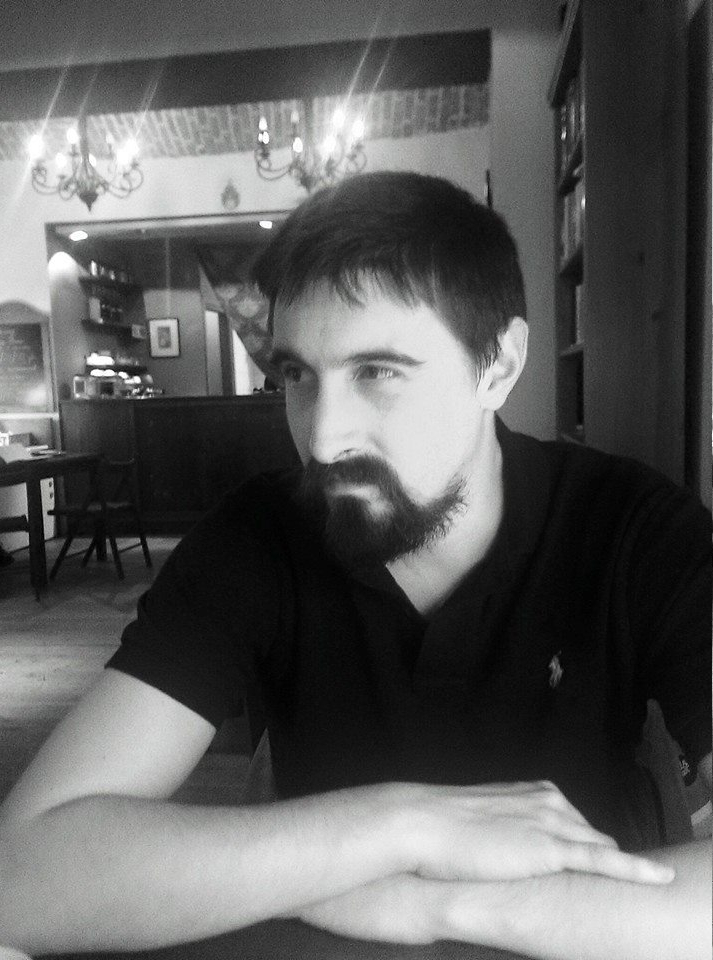# Optimal Control

 Web page Solid State NMR Web page Noise Resilience Web page Hessian Calculations

The Hamiltonian of a control system can be separated into two parts, one which is beyond the control of the experiment named the drift Hamiltonian,$\hat{\mathcal{H}}_0$, and all other parts of the Hamiltonian correspond to a specific controllable subsystem. In the case of magnetic resonance, the controllable part of the Hamiltonian consists of radio frequency control fields. In Hilbert space this Hamiltonian can be written as$\hat{\mathcal{H}}(t)= \hat{\mathcal{H}}_0 + \sum\limits_kc_k(t) \hat{\mathcal{H}}_k$

where$\hat{\mathcal{H}}_k$ are the control Hamiltonians and$c_k(t)$ are the time-dependent coefficients of the control Hamiltonians, corresponding to a control vector being a vector of amplitudes, exciting the system at this amplitude of radio frequency.

Investigation within this study is concerned with optimal control of NMR systems, specifically using the GRAPE algorithm . The task of optimal control reduces to finding the amplitudes within the control vector,$c_k(t)$, which control the evolution of the system from a defined initial state$\hat{\rho}(0)$ to a target state$\hat{\sigma}$ using an numerical optimization algorithm. Numerical optimization is concerned with finding and recognizing the minimum of an objective function.

State transfer quality is defined by the fidelity of the target state and the state of the system after a time$T$,$J=\Real\left\langle\hat{\sigma}\middle|\hat{\rho}(t=T)\right\rangle$ .$J=\Real \left\langle \hat{\sigma}\middle|\exp_{(0)}\middle[-i\int_0^T\left(\hat{\hat{\mathcal{H}}}(t)+i\hat{\hat{\mathcal{R}}}\right)\dd t\middle]\middle|\hat{\rho}(0)\right\rangle$

where the double hat,$\hat{\hat{A}}$, denotes the superoperator and$\exp_{(0)}$ is the time-ordered exponential .

Using the Hamiltonian separation outlined in earlier, we can define the optimality condition as$\frac{\partial J}{\partial c_k(t)}=0$

## References

 N. Khaneja, T. Reiss, C. Kehlet, T. Schulte-Herbruggen and S. J. Glaser, Journal of Magnetic Resonance 172, 296 (2005).
 I. Kuprov, Journal of Magnetic Resonance 233, 107 (2013).
 R. R. Ernst, G. Bodenhausen, and A. Wokaun, Principles of nuclear magnetic resonance in one and two dimensions, Vol. 14 (1991).